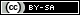# Arduino Function Generator (Part 2)

Last time, we looked at some Arduino code that we could use to generate some square waves.

The problem with the setup we’ve been looking at so far, is that we can only produce signals of one amplitude – equivalent to the HIGH logic level.

In order to be able to produce any other waveforms we’ll need to be able to produce a variety of different output voltages. Although the PWM method we looked at last time gives us a way to do this, it’s not suitable for producing variable waveforms – as it’s time-based. We can see this, if we try to use PWM to produce a triangular waveform: and view the output with an oscilloscope.

```void setup()
{
pinMode(11, OUTPUT);
}

void loop()
{
for (int i=0; i<0; i--)
{
analogWrite(11, i);
delay(1);
}
}```If we hook that up to our oscilloscope – it looks like this:It’s not too easy to see that as a still image – so here’s a video clip of the same thing…

That’s clearly not really what we wanted: so we need to do something differently.

The solution to this problem, is to use some kind of digital-to-analogue converter (or DAC). To begin with, let’s build our own…Or, if you prefer, in schematic…This circuit is an 8-bit DAC known as an R-2R resistor ladder network. Each of our eight bits contributes to the resultant output voltage. If all 8-bits are HIGH – then the output is approximately equal to the reference voltage… If we switch the most-signifincant bit to LOW – then we get approximately half of that voltage.

More precisely, for an 8-bit DAC if all 8-bits are HIGH – then we get 255/256th of the reference voltage. Switching the most-significant bit to LOW gives us 127/256th of the reference voltages. More generally for any bit value x, between 0 & 255, our DAC will us x/256th of the reference voltage.

There’s a short article on wikipedia explaining R-2R DACs in a little more detail, if you want to know more…

Okay, now that we’ve built our circuit – let’s do something with it.

We’ll start by producing a triangular waveform…

```void setup()
{
pinMode(0, OUTPUT);
pinMode(1, OUTPUT);
pinMode(2, OUTPUT);
pinMode(3, OUTPUT);
pinMode(4, OUTPUT);
pinMode(5, OUTPUT);
pinMode(6, OUTPUT);
pinMode(7, OUTPUT);
}

void loop()
{
for (int i=0;i<255;i++)
{
PORTD=i;
}

for (int i=255;i>0;i--)
{
PORTD=i
}
}```Note that here we’re using `PORTD` instead of setting the state of the individual output pins one at a time. This is much faster, and it (critically) ensures that all of the pins change at the same time – and that’s important given we want to switch smoothly though digital values to create a smoothly changing analogue voltage.

`PORTD` is port register D. Our use of it here works because it maps to the 0-7 digital pins (giving us 8-bits); and writing either a binary or decimal value to the register – we control all 8 pins in one operation. For example, if we assigned it the decimal value 123 (which equates to `B01111011`) would set pins 6, 5, 4, 3, 1, & 0 to HIGH… Now the problem is that pin 0 is used for communicating serial data – so using `PORTD` means that we can’t use any serial communications whilst code it running. It is, however, the only way to manipulate 8 output pins simultaneously (and we didn’t need serial communications for this application anyway).

We can modify our code very easily, to produce a saw-tooth waveform – by simply commenting out (or otherwise removing) the second of the two `for` loops.

Note that if we want to drive anything significant (even something as lowly as an LED) we need add a transistor into the circuit, like this:Finally, on to a sine wave. We’ll need to pre-compute some values for the output voltages over time (the arduino just isn’t quite fast enough to be able to this in real-time, in a situation quite as time-sensitive as producing a waveform).

A sine wave has a cycle of 2π radians – so to produce a sine wave with 256 time-steps per cycle, we just need to calculate y=sin((x/255)*2π) for each point value of x. Since the sine function gives us values between 1 and -1; and we want values between 0 and 255, the simplest way to do this is to multiply the `float` value by 128 – giving us a value between -128 & +128; and then add 128 – to give us the correct range.

We could do this off-line; but that’s a bit tedious – so we’ll get the arduino to do this for us, in the `setup()` phase, storing the results in a global array. Then all we need to do in our main loop, is cycle through the array.

```int sine;
void setup()
{
pinMode(0, OUTPUT);
pinMode(1, OUTPUT);
pinMode(2, OUTPUT);
pinMode(3, OUTPUT);
pinMode(4, OUTPUT);
pinMode(5, OUTPUT);
pinMode(6, OUTPUT);
pinMode(7, OUTPUT);
float x;
float y;
for(int i=0;i<255;i++)
{
x=(float)i;
y=sin((x/255)*2*PI);
sine[i]=int(y*128)+128;
}
}

void loop()
{
for (int i=0;i<255;i++)
{
PORTD=sine[i];
delayMicroseconds(10);
}
}```If we run that code, with our DAC, and have a look at that on our oscilloscope we see that we do indeed have something that looks quite a lot like a sine wave.That’s all for this part, next time we’ll have a look at varying the frequency of our waveforms; and look at a more compact and accurate way to do the digital-to-analogue conversion, using a dedicated IC…

## 3 thoughts on “Arduino Function Generator (Part 2)”

1.Ilja Bobkevic says:

Thank you very much for publishing this. It was very helpful!

2.Cayce says:

Great tutorial ! Looking forward to part 3 !!!!

1.AJP says:

Thanks. By the way, in case you missed it, part three can be found here: https://www.auctoris.co.uk/2012/04/13/arduino-function-generator-part-3/

This site uses Akismet to reduce spam. Learn how your comment data is processed.